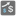• # Why doesn't this code work?

## Question related to missionBigger Price

My first attempt at solving the Bigger Price task, I did this:

```def bigger_price(limit: int, data: list) -> list:
v1 = 0
l1 = []
while len(l1)< limit:
for i in range(len(data)):
if v1 <= data[i].get("price", data[i]):
v1 = data[i].get("price", data[i])
l1.insert(0, data[i])

return l1
```

It wouldn't accept despite getting the correct results, as far as I can tell. I then did it another way:

```def bigger_price(limit: int, data: list) -> list:
l1 = sorted(data, key=lambda x: x['price'], reverse = True)

return l1[0:limit]
```

And that passed. Why?

PS ignore indentation issue. I don't know how to indent correctly on the forums.11